StatLect

# Marginal probability density function

Consider a continuous random vector, whose entries are continuous random variables.

Each entry of the random vector has a univariate distribution described by a probability density function (pdf). This is called marginal probability density function, to distinguish it from the joint probability density function, which depicts the multivariate distribution of all the entries of the random vector.## Definition

A more formal definition follows.

Definition Letbecontinuous random variables forming acontinuous random vector. Then, for each, the pdf of the random variable, denoted by, is called marginal probability density function.

## What you need to know

Before explaining how to derive the marginal pdfs from the joint pdf, let us revise the basics of pdfs.

### Pdf

The probability density function of a variableis a functionsuch that the probability thatwill take a value in the intervalis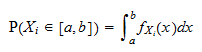for any interval### Joint pdf

The joint probability density function of the vectoris a function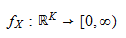such that the probability thatwill take a value in the interval, simultaneously for all, is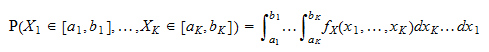for any hyper-rectangle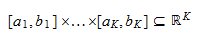## How to derive the marginal pdf

The marginal probability density function ofis obtained from the joint pdf as follows: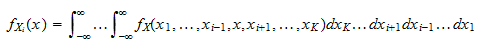In other words, to compute the marginal pdf of, we integrate the joint pdf with respect to all the variables except.

## Example

Letbe acontinuous random vector having joint pdf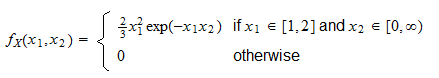To derive the marginal probability density function of, we integrate the joint pdf with respect to.

When, then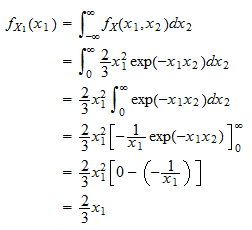When, thenTherefore, the marginal probability density function ofis## Other examples

On the following pages you can find other examples and detailed derivations:

## More details

Marginal probability density functions are discussed in more detail in the lecture on Random vectors.

## Keep reading the glossary

Previous entry: Marginal distribution function

Next entry: Marginal probability mass function